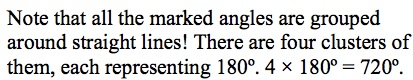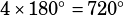Hello, I am having trouble with the explanation for Angles, Triangles, and Polygons problem #2 on page 343. To get the answer to this problem, I multiplied 180*4, as there are four sets of angles that each make up a straight line, and the question asks for their sum. The solution seems to be getting at this, but instead of saying “180° x 4=720°” it says “180°.4 x 180° = 720°”. (I can send you a picture if you want.) Is this a typo, or am I misunderstanding?That’s not a typo—the period you see after 180° is the end of the sentence. Then the next sentence is all math:. Does that help?